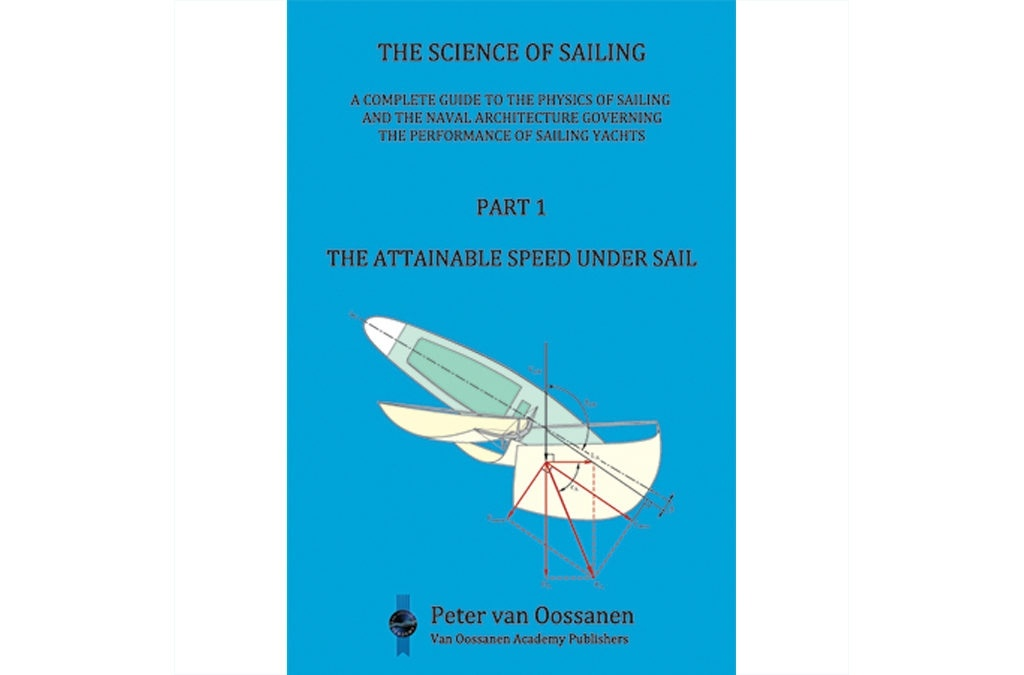# The Science of Sailing Part 1 "The Attainable Speed Under sail"# The Science of Sailing Part 1 "The Attainable Speed Under sail"Comenta y valora este libro

### FICHA TÉCNICA

art 1 consists of Chapters 1 through 4. Chapter 1 is an introduction to the collected work. Chapter 2 provides a review of basic notions and considerations which readers with a formal training in naval architecture and in aero and hydrodynamics, may skip over. This chapter describes first of all the scientific method which lies at the base of all science since the days of Isaac Newton. The nature of mathematical formulae and how these are typically derived is then addressed followed by a summary of the basic mathematical functions utilized to form such formulae. The building of mathematical models and algorithms is described, followed by a portrayal of the physical quantities we work with, their dimension, and the units we have given to them. The concept and use of non-dimensional coefficients and numbers is reviewed and a description is given of the properties of air and water  the two mediums in which a sailing vessel, at the interface between the two, operates. Chapters 3 and 4 provide a description of the basic speed-producing factors for sailing craft that can be derived from setting the resultant aerodynamic force equal and opposite to the resultant hydrodynamic force. These two overall forces must be in equilibrium when a wind-driven vessel is sailing along a straight course at constant speed in a steady wind, when the sails are efficiently trimmed and the rudder is set at a steady helm angle. Some important conclusions can be derived from this basic equilibrium condition and these two chapters present these, utilizing some assumptions which, in later chapters, are further validated. The mathematical treatment of Chapter 4 is particularly developed for the purpose of showing the degree to which the speed of wind-driven craft is dependent on the aerodynamic lift and drag forces acting on the above-water parts of the vessel and the hydrodynamic lift and drag forces acting on the below-water hull and appendages. This chapter sets the scene for the following chapters that deal with the many aspects of fluid-d....